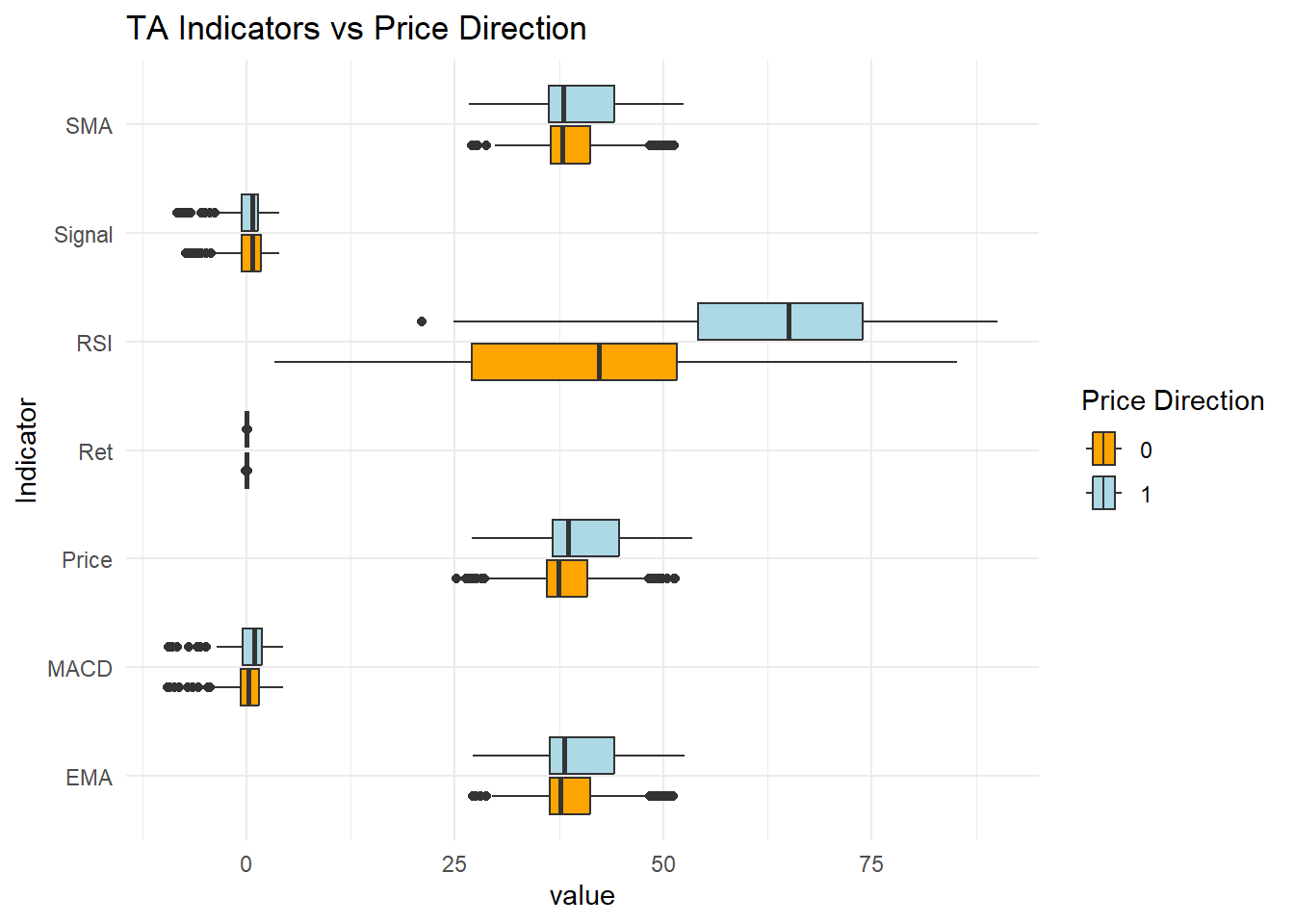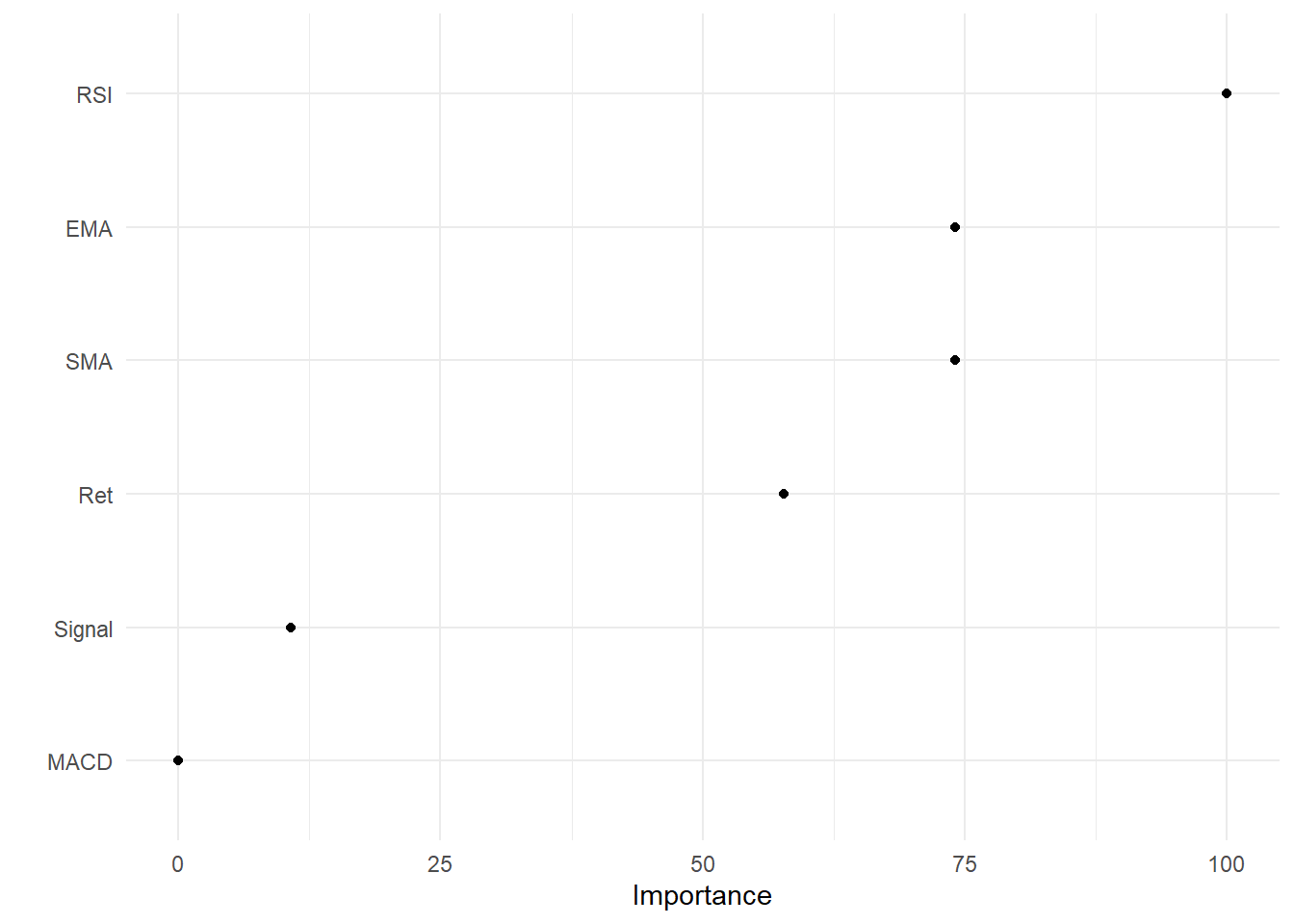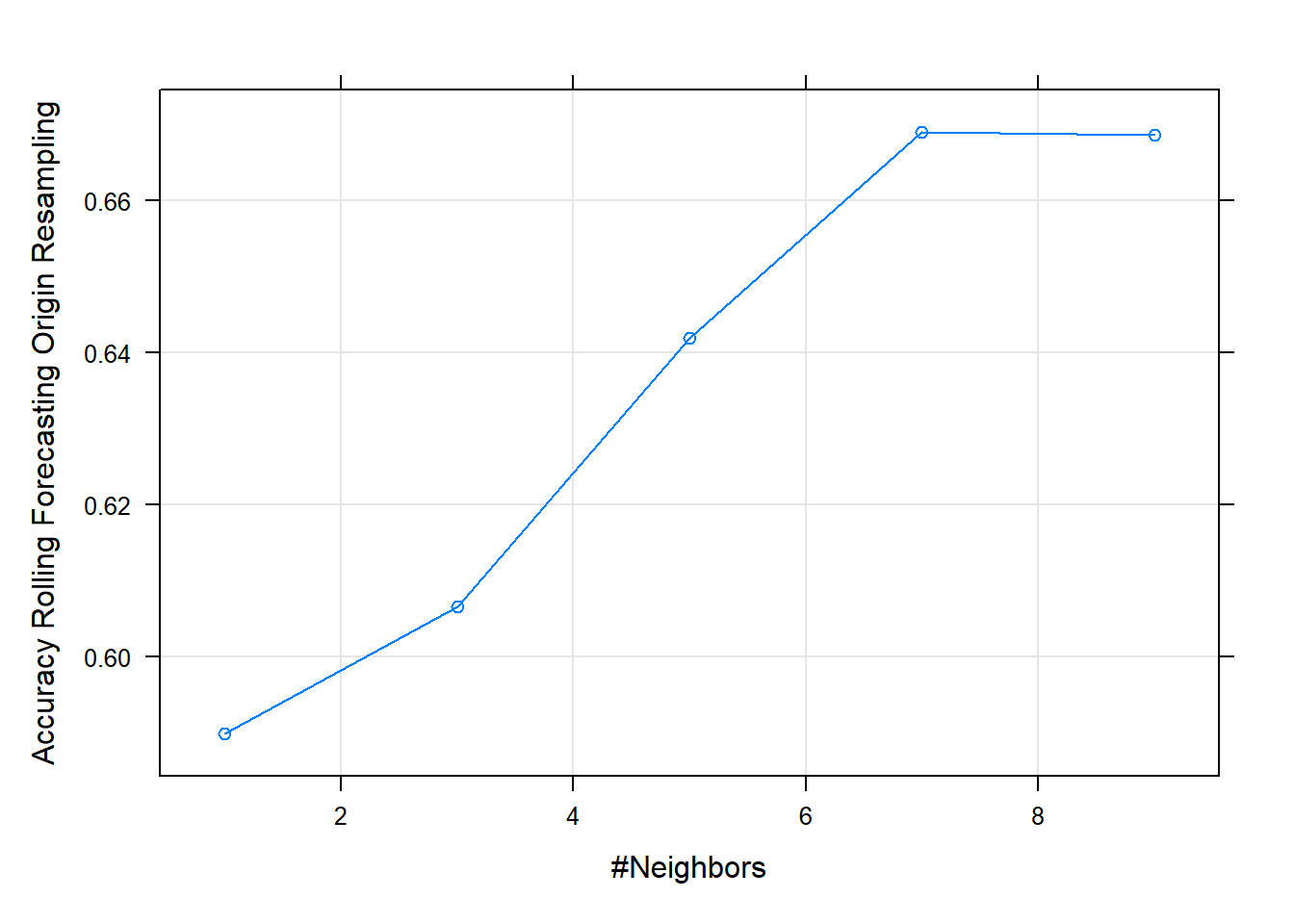## 14.3 Forecasting Stock Price Movement using ML

Topic-7 provided an introduction to using R for creating Technical Indicators for traded assets. This example uses selected indicators to predict the direction of the price movement, i.e., if the prices will be higher or lower the next day. Various studies have evaluated ML methods to predict the price direction or return, for example, examined indicators like SMA, EMA etc on various forecast horizons and window lengths.

• The example will use the closing prices of a stock (BHP in this case) to create the following Technical Indicators

• Lag of 5 Days Simple Moving Average : Moving averages are the simplest among all indicators: they show the average price level for you on a rolling basis.

• Lag 5 Days Exponential Moving Average: The difference between SMA and EMA is that SMA weighs all candles equally whereas EMA gives exponential weights – hence the name: it overweights recent days to previous ones.

• MACD: Moving Average Convergence Divergence: Uses EMA of 26 and 12 days. Designed to reveal changes in strength, direction, momentum and duration of a trend in stock price.

• Lag of Log Returns

• Lag of RSI: Momentum oscillator that measures the speed and change of price movements. Ranges from zero to 100. Can be used to identify general trends.

• The response (dependent) variable is constructed based on the previous day(s) prices. Here we use the following indicator:

$\begin{equation} 1 \rightarrow P_{t}\geq P_{t-5}\\ 0\, otherswise \tag{14.3} \end{equation}$

### 14.3.1 Data & Indicators

library(quantmod)
library(TTR)
library(xts)  #required as the data was saved as an xts object
d_bhp = d_bhp$BHP.AX.Close #select close prices colnames(d_bhp) = "Price" # SMA sma5 = lag(SMA(d_bhp, n = 5)) #notice the use of the lag function to take lagged values # EMA ema5 = lag(EMA(d_bhp, n = 5)) # MACD macd1 = lag(MACD(d_bhp)) # RSI rsi1 = lag(RSI(d_bhp, 5)) # log returns ret1 = lag(dailyReturn(d_bhp, type = "log")) # price director indicator dir = ifelse(d_bhp$Price >= lag(d_bhp$Price, 5), 1, 0) #direction variable compared to 5 day before price • Combine all the indicators and response variable in a data frame d_ex1 = cbind(dir, ret1, sma5, ema5, macd1, rsi1) # change column names colnames(d_ex1) = c("Direction", "Ret", "SMA", "EMA", "MACD", "Signal", "RSI") ### 14.3.2 Visualise the data • Using the quantmod package chartSeries(d_bhp, theme = "white", name = "BHP Closing Prices and Indicators") addTA(d_ex1[, 1], col = 1, legend = "Direction") #Direction addTA(d_ex1[, -1], on = NA, col = rainbow(6), legend = as.character(colnames(d_ex1[, -1])))Figure 14.1: Direction and Technical Indicators • The above graph can be improved using ggplot2 library(tidyr) library(ggplot2) # create a dataset and convert data to long d_plot = merge.xts(d_bhp, d_ex1) # remove NAs and then convert to long d_plot = na.omit(d_plot) # convert to dataframe d_plot = data.frame(Date = index(d_plot), coredata(d_plot)) d_plot_long = pivot_longer(d_plot, -c(Date, Direction), values_to = "value", names_to = "Indicator") # change direction to a factor d_plot_long$Direction = as.factor(d_plot_long$Direction) (p2_ex = ggplot(d_plot_long, aes(Date, value, color = Indicator)) + geom_path(stat = "identity") + facet_grid(Indicator ~ ., scale = "free") + theme_minimal())Figure 14.2: Indicators and Prices (ggplot2) • The above is a line chart of indicators and prices. We can also create a box plot to visualise the variance in the values relative to the direction p2_ex = ggplot(d_plot_long, aes(value, Indicator, fill = Direction)) + geom_boxplot() p2_ex + theme_minimal() + labs(title = "TA Indicators vs Price Direction") + scale_fill_manual(name = "Price Direction", values = c("orange", "lightblue"))Figure 14.3: Box Plot of Indicators • Some differences per category can be noticed ### 14.3.3 Using Logistic Regression • 70:30 data split • Time series sampling • Stratified sampling will not keep the time order and hence its avoided # remove NAs d_ex1 = na.omit(d_ex1) # convert to data frame d_ex1 = as.data.frame(d_ex1) # convert direction to a factor for classification d_ex1$Direction = as.factor(d_ex1$Direction) idx1 = c(1:round(nrow(d_ex1) * 0.7)) #create index for first 70% values to be in the testing set d_train1 = d_ex1[idx1, ] #training set d_test1 = d_ex1[-idx1, ] #testing set Training Setup • Time series cross validate for resampling: 250 day window 30 days for prediction (this can be changed) • Data preprocessing is conducted to normalise the scale of the values • glm method with binomial family for the binary classification library(caret) set.seed(999) # control cntrl1 = trainControl(method = "timeslice", initialWindow = 250, horizon = 30, fixedWindow = TRUE) # preprocesing prep1 = c("center", "scale") # logistic regression logit_ex1 = train(Direction ~ ., data = d_train1, method = "glm", family = "binomial", trControl = cntrl1, preProcess = prep1) logit_ex1 #final model accuracy Generalized Linear Model 434 samples 6 predictor 2 classes: '0', '1' Pre-processing: centered (6), scaled (6) Resampling: Rolling Forecasting Origin Resampling (30 held-out with a fixed window) Summary of sample sizes: 250, 250, 250, 250, 250, 250, ... Resampling results: Accuracy Kappa 0.7324731 0.4658076 summary(logit_ex1$finalModel)  #summary of the final model

Call:
NULL

Deviance Residuals:
Min        1Q    Median        3Q       Max
-2.43299  -0.63007  -0.04745   0.57603   2.49565

Coefficients:
Estimate Std. Error z value Pr(>|z|)
(Intercept)  -0.05772    0.13429  -0.430    0.667
Ret          -0.82494    0.18720  -4.407 1.05e-05 ***
SMA         -20.20397    3.70217  -5.457 4.83e-08 ***
EMA          20.05707    3.67374   5.460 4.77e-08 ***
MACD          0.50610    0.73203   0.691    0.489
Signal       -0.94914    0.68541  -1.385    0.166
RSI           2.14092    0.30037   7.128 1.02e-12 ***
---
Signif. codes:  0 '***' 0.001 '**' 0.01 '*' 0.05 '.' 0.1 ' ' 1

(Dispersion parameter for binomial family taken to be 1)

Null deviance: 601.61  on 433  degrees of freedom
Residual deviance: 348.17  on 427  degrees of freedom
AIC: 362.17

Number of Fisher Scoring iterations: 5
• Variable importance
• In the case of multiple predictor variables, we want to understand which variable is the most influential in predicting the response variable.
library(vip)
vip(logit_ex1, geom = "point") + theme_minimal()Figure 14.4: Variable Importance

Predictive Accuracy

• ML models should be checked for predictive accuracy on the test set
• caret provides predict function to create predictions
• These predictions can be assessed based on the confusion matrix

Confusion Matrix - When applying classification models, we often use a confusion matrix to evaluate certain performance measures.

• A confusion matrix is simply a matrix that compares actual categorical levels (or events) to the predicted categorical levels.

• Prediction of the right level; refer to this as a true positive.

• Prediction of a level or event that did not happen this is called a false positive (i.e. we predicted a customer would redeem a coupon and they did not).

• Alternatively, when we do not predict a level or event and it does happen that this is called a false negative (i.e. a customer that we did not predict to redeem a coupon does).

• Let’s predict using the final model and assess using the confusion matrix.

pred1 = predict(logit_ex1, newdata = d_test1)  #prediction on the test data
confusionMatrix(data = pred1, reference = d_test1$Direction) Confusion Matrix and Statistics Reference Prediction 0 1 0 52 26 1 16 92 Accuracy : 0.7742 95% CI : (0.7073, 0.8321) No Information Rate : 0.6344 P-Value [Acc > NIR] : 2.959e-05 Kappa : 0.5279 Mcnemar's Test P-Value : 0.1649 Sensitivity : 0.7647 Specificity : 0.7797 Pos Pred Value : 0.6667 Neg Pred Value : 0.8519 Prevalence : 0.3656 Detection Rate : 0.2796 Detection Prevalence : 0.4194 Balanced Accuracy : 0.7722 'Positive' Class : 0  • The model provides decent accuracy which is higher than the No Information Rate and also statistically significant. There are other analyses which can be conducted on logistic regression, for example, ROC (Receiver Operating Characteristic) curve analysis for the Area Under the Curve. ### 14.3.4 Using K-NN • This section will implement the previous model using k-nearest neigbour algorithm using the caret package • Then we will use the same split as the logistic regression • We are selecting the model based on Accuracy & Kappa • Accuracy: Accuracy measures the overall correctness of the classifier $$\frac{(TN+TP)}{Total}$$. Objective: maximise • Kappa (Cohen’s Kappa): Its like Accuracy but it is normalised at the baseline of random chance on the dataset. It compares observed accuracy to expected accuracy. • A grid search is used to search for an optimal value for k set.seed(999) grid1 = expand.grid(k = seq(1, 10, by = 2)) #to search from k=1 to k=10 knn_ex1 = train(Direction ~ ., data = d_train1, method = "knn", tuneGrid = grid1, trControl = cntrl1, preProcess = prep1) • View the fit plot(knn_ex1) #may suggest using a wider gridFigure 14.5: Grid plot knn_ex1 k-Nearest Neighbors 434 samples 6 predictor 2 classes: '0', '1' Pre-processing: centered (6), scaled (6) Resampling: Rolling Forecasting Origin Resampling (30 held-out with a fixed window) Summary of sample sizes: 250, 250, 250, 250, 250, 250, ... Resampling results across tuning parameters: k Accuracy Kappa 1 0.5898925 0.1554741 3 0.6066667 0.1834679 5 0.6419355 0.2500894 7 0.6690323 0.3194890 9 0.6686022 0.3209926 Accuracy was used to select the optimal model using the largest value. The final value used for the model was k = 7. Variable importance can’t be obtained here as KNN does not produce a model Predictive Accuracy Let’s predict using the final model and assess using the confusion matrix. pred2 = predict(knn_ex1, newdata = d_test1) #prediction on the test data confusionMatrix(data = pred2, reference = d_test1$Direction)
Confusion Matrix and Statistics

Reference
Prediction  0  1
0 50 50
1 18 68

Accuracy : 0.6344
95% CI : (0.5608, 0.7037)
No Information Rate : 0.6344
P-Value [Acc > NIR] : 0.5330295

Kappa : 0.2833

Mcnemar's Test P-Value : 0.0001704

Sensitivity : 0.7353
Specificity : 0.5763
Pos Pred Value : 0.5000
Neg Pred Value : 0.7907
Prevalence : 0.3656
Detection Rate : 0.2688
Detection Prevalence : 0.5376
Balanced Accuracy : 0.6558

'Positive' Class : 0

• A comparison betweek Logistic Regression and KNN can be made based on their predictive accuracy.

• The two models can also be compared based on their resampling results.

• The random number seeds should be initialised to the same value for resampling comparison.

• The following code compares the model fits.

resamp1 = resamples(list(logit = logit_ex1, knn = knn_ex1))

summary(resamp1)

Call:
summary.resamples(object = resamp1)

Models: logit, knn
Number of resamples: 155

Accuracy
Min.   1st Qu.    Median      Mean   3rd Qu.      Max. NA's
logit 0.4666667 0.6666667 0.7333333 0.7324731 0.8000000 0.9000000    0
knn   0.4333333 0.5666667 0.6666667 0.6690323 0.7666667 0.8666667    0

Kappa
Min.   1st Qu.    Median      Mean   3rd Qu.      Max. NA's
logit  0.1208791 0.3333333 0.4666667 0.4658076 0.5838144 0.7887324    0
knn   -0.1860465 0.1558442 0.3243243 0.3194890 0.4666667 0.7235023    0
bwplot(resamp1, metric = "Accuracy")Figure 14.6: Resample Accuracy comparison

• The graph provides a comparison of the overall accuracy of the model (not the predictions).

### References

Shynkevich, Yauheniya, T. M. McGinnity, Sonya A. Coleman, Ammar Belatreche, and Yuhua Li. 2017. “Forecasting Price Movements Using Technical Indicators: Investigating the Impact of Varying Input Window Length.” Neurocomputing 264: 71–88. https://doi.org/https://doi.org/10.1016/j.neucom.2016.11.095.